# Physics 2102 Jonathan Dowling Physics 2102 Lecture 02

• Slides: 14Physics 2102 Jonathan Dowling Physics 2102 Lecture 02: THU 21 JAN Electric Fields I Charles-Augustin de Coulomb (1736 -1806)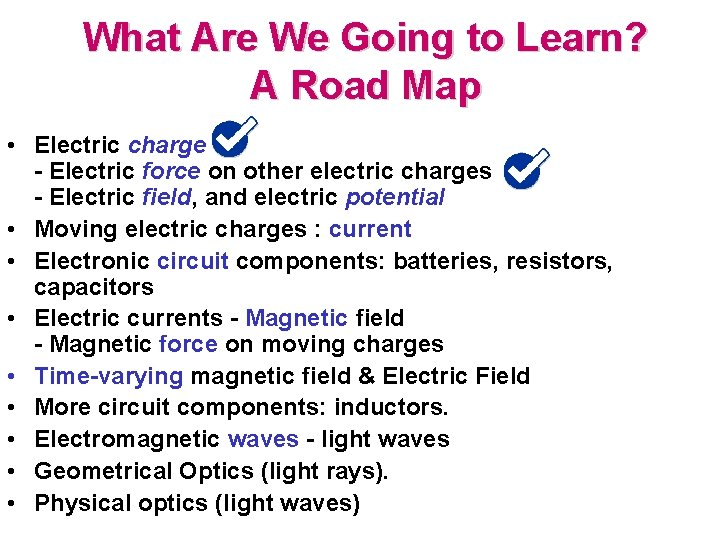What Are We Going to Learn? A Road Map • Electric charge - Electric force on other electric charges - Electric field, and electric potential • Moving electric charges : current • Electronic circuit components: batteries, resistors, capacitors • Electric currents - Magnetic field - Magnetic force on moving charges • Time-varying magnetic field & Electric Field • More circuit components: inductors. • Electromagnetic waves - light waves • Geometrical Optics (light rays). • Physical optics (light waves)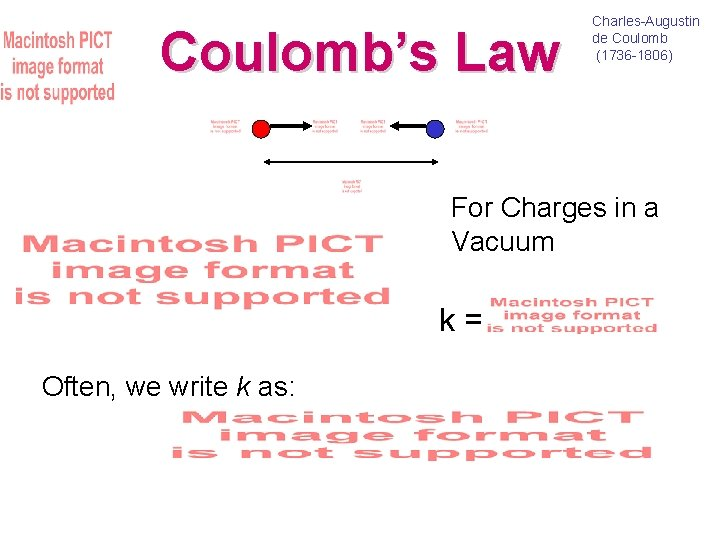Coulomb’s Law Charles-Augustin de Coulomb (1736 -1806) For Charges in a Vacuum k = Often, we write k as: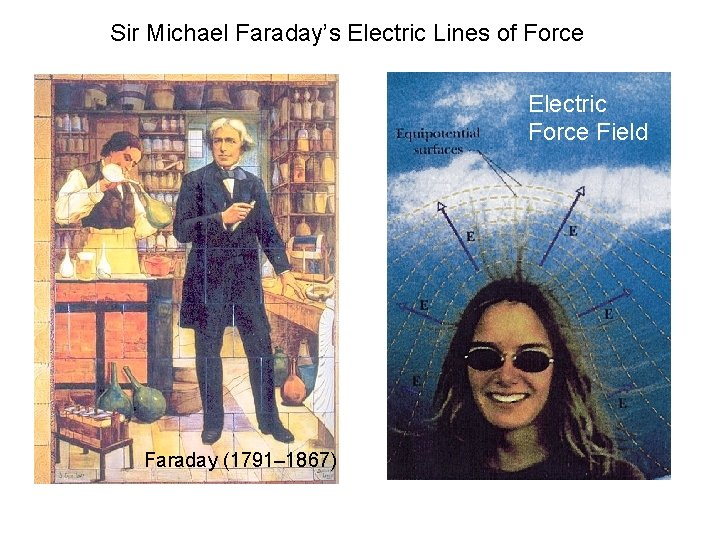Sir Michael Faraday’s Electric Lines of Force Electric Force Field Faraday (1791– 1867)E-Field is E-Force Divided by ECharge Definition of Electric Field: E-Force on Charge +q 1 –q 2 P 1 P 2 E-Field at Point –q 2 P 1 P 2 Units: F = [N] = [Newton] ; E = [N/C] = [Newton/Coulomb]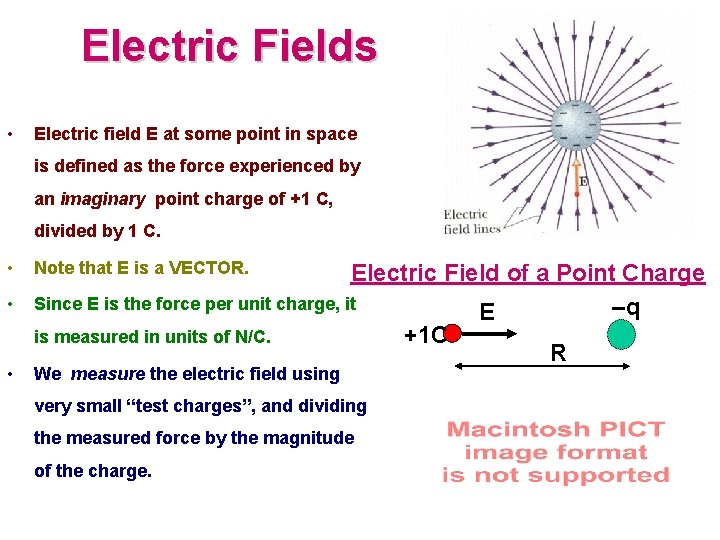Electric Fields • Electric field E at some point in space is defined as the force experienced by an imaginary point charge of +1 C, divided by 1 C. • • • Note that E is a VECTOR. Electric Field of a Point Charge Since E is the force per unit charge, it –q E +1 C is measured in units of N/C. R We measure the electric field using very small “test charges”, and dividing the measured force by the magnitude of the charge.Superposition of F and E • Question: How do we figure out the force or field due to several point charges? • Answer: consider one charge at a time, calculate the field (a vector!) produced by each charge, and then add all the vectors! (“superposition”) • Useful to look out for SYMMETRY to simplify calculations! • If you never learned to add vectors in 2101 you’ll be in serious trouble in 2102! See online review http: //phys. lsu. edu/~jdowling/ PHYS 21024 SP 10/Vectors. pdf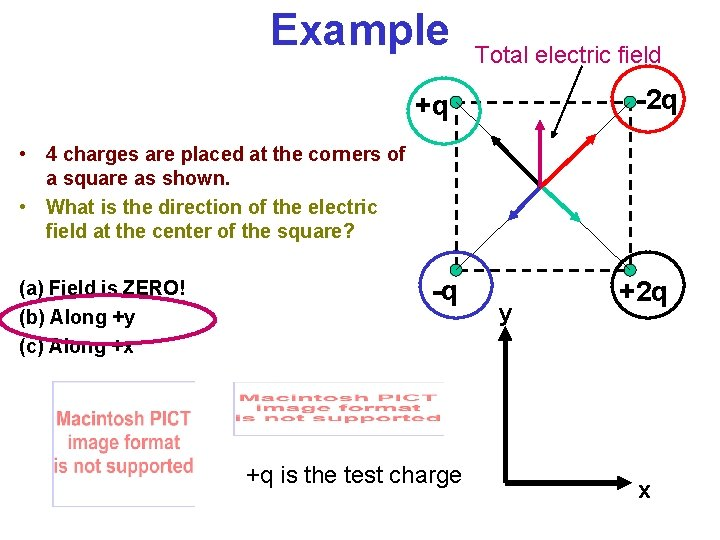Example Total electric field -2 q +q • 4 charges are placed at the corners of a square as shown. • What is the direction of the electric field at the center of the square? (a) Field is ZERO! (b) Along +y (c) Along +x -q +q is the test charge y +2 q x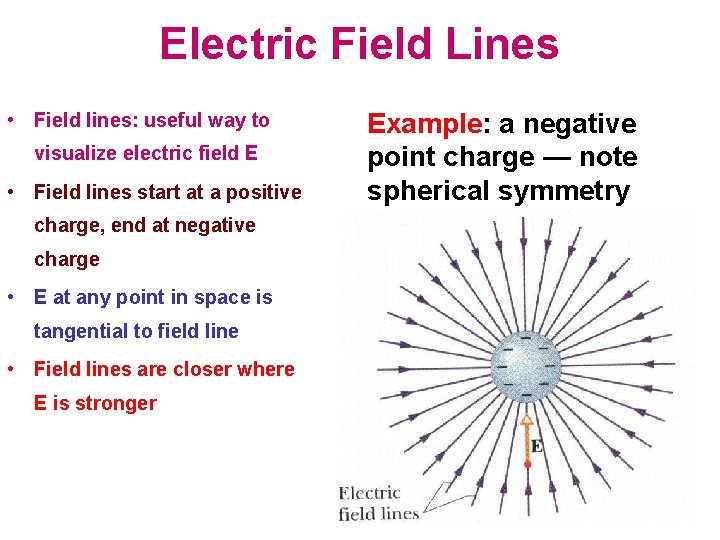Electric Field Lines • Field lines: useful way to visualize electric field E • Field lines start at a positive charge, end at negative charge • E at any point in space is tangential to field line • Field lines are closer where E is stronger Example: a negative point charge — note spherical symmetryDirection of Electric Field Lines E-Field Vectors Point Away from Positive Charge — Field Source! E-Field Vectors Point Towards Negative Charge — Field Sink!Electric Field of a Dipole • Electric dipole: two point charges +q and –q separated by a distance d • Common arrangement in Nature: molecules, antennae, … • Note axial or cylindrical symmetry • Define “dipole moment” vector p: from –q to +q, with magnitude qd Cancer, Cisplatin and electric dipoles: http: //chemcases. com/cisplat 01. htm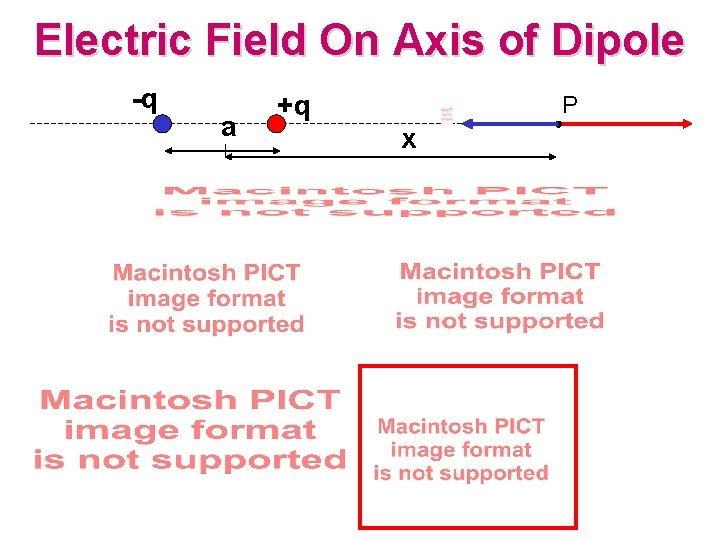Electric Field On Axis of Dipole -q a +q P x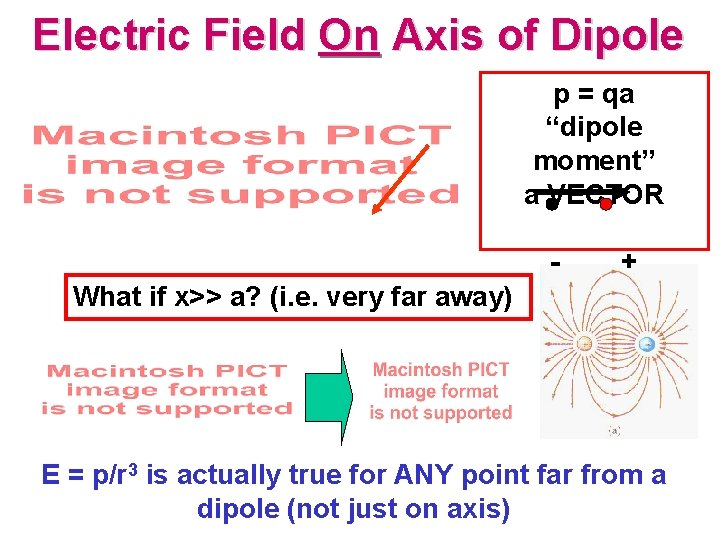Electric Field On Axis of Dipole p = qa “dipole moment” a VECTOR - + What if x>> a? (i. e. very far away) E = p/r 3 is actually true for ANY point far from a dipole (not just on axis)